S k i l l
i n
A R I T H M E T I C

Lesson 22

# COMPARING FRACTIONS

In this Lesson, we will answer the following:

1. How can we compare fractions with the same numerator?
2. How can we compare fractions with the same denominator?
3. How can we find the lowest common multiple of two numbers?
4. When is the LCM of two numbers simply their product?
5. How can we compare fractions when the numerators and denominators are different?

Section 2

6. How can we know the ratio of any two fractions?
7. How can we know whether a fraction is more than or less than ½?

 1. How can we compare fractions with the same numerator?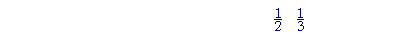The larger the denominator, the smaller the fraction.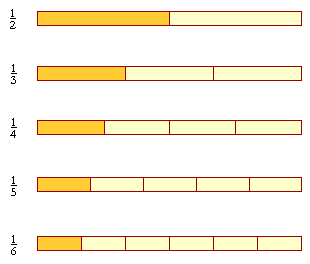In each of these, the numerator is the same:  1.  As the denominators

 get larger, then the fraction -- the size of each piece -- gets smaller. 16 is
 smaller than 15 .

For, in terms of ratios, if the whole is in 6 pieces, then 1 of 6 pieces --

 16 --  is smaller than 1 of 5 pieces: 15 .

Further, since one-sixth is smaller than one-fifth, then

 2 6 is smaller than 25 .

Three will be smaller than three:

 3 6 is smaller than 35 .

And so on.

When the numerators are the same, then the larger the denominator, the smaller the fraction.

In terms of ratios, then the ratio of 1 to 2, for example, is greater than the ratio of 1 to 3: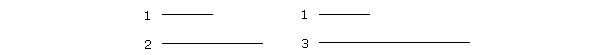When we compare 1 with 2, it appears greater than when we compare it with 3.

 2. How can we compare fractions with the same denominator?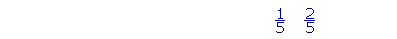The larger the numerator, the larger the fraction.25 is larger than 15 .  For we're counting units -- 15 's.
 35 is larger than 25 .
 45 is larger than 35 .

And so on, when the denominators are the same.

 Equivalently, 3 out of 5  -- 35 -- is more than 2 out of 5: 25 .

As for ratios, we say that the ratio of 2 to 5 is smaller than the ratio of 3 to 5:2, when compared with 5, appears smaller than 3 when compared with 5.

Example 1.   Arrange these from smallest to largest:

 57 49 47
 Solution.  We must compare them in pairs. 49 and 47 have the same

numerator; therefore

 49 is smaller than 47 .
 57 and 47 have the same denominator; therefore
 57 is larger than 47 .

The sequence is

 49 47 57 .

 Example 2.   Which is smaller, 1 10 or 29 ?
 Answer.  Since 1 10 is smaller than 19 , then it is surely smaller than 29 .

Lowest common multiple

We will now consider fractions in which the numerators and denominators are different.  But first, we must learn about the lowest common multiple.

Here are the multiples of 6:

6,  12,  18,  24, . . .

And here are the multiples of 8:

8,  16,  24,  32, . . .

24 is a common multiple of 6 and 8.  It is their lowest common multiple, which we abbreviate as the LCM.The LCM is the first time that the multiples of 6 meet the multiples of 8.

 3. How can we find the lowest common multiple of two numbers? Go through the multiples of the larger numberuntil you come to a multiple of the smaller number.

Example 3.   Find the LCM of 9 and 12.

Solution.   Go through the multiples of 12 until you come to a multiple of 9.

12,  24,  36.

36 is the first multiple of 12 that is also a multiple of 9.  36 is their LCM.

Example 4.   Find the LCM of 2 and 8.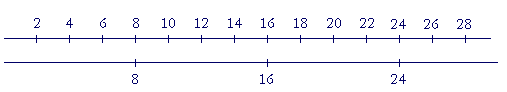8 itself is their LCM.

When the larger number is itself a multiple of the smaller number, then the larger number itself is their LCM.

Example 5.   Find the LCM of 5 and 20.

Solution.   20 is their LCM.

Now the product of two numbers will always be a common multiple.  The product of 6 and 4, for example, is 24, and 24 is a common multiple -- but it is not their lowest common multiple.  Their lowest common multiple is 12.

 4. When is the LCM of two numbers simply their product? When 1 is their only common divisor.

Example 6.   What is the LCM of 6 and 7?

Answer.  1 is their only common divisor. Therefore their LCM is 6 × 7 = 42.

(1 is a common divisor of every pair of numbers, but some pairs have 1 as their only common divisor.  6 and 7 are such a pair.)

Example 7.   What is the LCM of 8 and 12?

Answer.  24.  Their LCM is not 8 × 12, because 8 and 12 have common divisors besides 1;  for example, 4.

(To find the LCM from prime factors, see Lesson 32.)

We will now see how to compare any two fractions.

 5. How can we compare fractions when the numerators and denominators are different?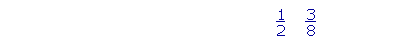Change them to equivalent fractions with the same denominator. (Lesson 21.) As the common denominator, choose their LCM. Then, compare the numerators.

 Example 8.   Which is larger, 12 or 38 ?

Answer.  Make a common denominator.  Choose the LCM of 2 and 8 -- which is 8 itself.  (Example 4, above.)

 We will change 12 to an equivalent fraction with denominator 8:
 12 = 48 .

 The denominators are now the same, and we can compare 48 with 38 .

We see:

 48 is larger than 38 .

That is,

 12 is larger than 38 .

 Example 9.   Which is larger, 34 or 2532 ?

Answer.  Again, we will make the denominators the same, and then compare the numerators.  As a common denominator, we will choose the LCM of 4 and 32, which is 32 itself.

 We will express 34 with denominator 32. On multiplying both

terms by 8,

 34 = 2432 .
 We are now comparing 34 , or
 2432 with 2532 .
 25 is larger than 24; therefore 2532 is larger than 34 .

 Example 10.   Which is larger, 56 or 79 ?

Answer.  As a common denominator, choose the LCM of 6 and 9.

Answer.  Choose 18.

 56 = 1518 , 79 = 1418 .
 To change 56 , we multiplied both terms by 3.  To change 79 , we multiplied

both terms by 2.

We choose a common multiple of the denominators, because we change denominators by multiplying them.

 Now, 15 is larger than 14. Therefore, 56 is larger than 79 .

Adding fractions (as we will see in Lesson 24) involves the same technique as comparing them, because the denominators -- the units -- must be the same.  For example,

 56 + 79 = 1518 + 1418 = 2918 .

At this point, please "turn" the page and do some Problems.

or

Continue on to the next Section.

www.proyectosalonhogar.com